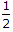# Civil Engineering - UPSC Civil Service Exam Questions

26.

Consider the following statements :
Soft varieties of pig iron are also known as
1. foundry pig
2. grey pig
3. forge pig
4. mottled pig.
Of these statements :

 A. 1, 2, 3 and 4 are correct B. 1 alone is correct C. 1 and 2 are correct D. 3 and 4 are correct

Explanation:

No answer description available for this question. Let us discuss.

27.

A turn-table on railways is used for

 A. preventing the lateral movement of wheels B. reversing the direction of the engine C. reducing the damage to the rails D. reducing the accidents

Explanation:

No answer description available for this question. Let us discuss.

28.

Consider the following statements regarding use of mechanical vibrator :
1. It can be used for small as well as large volumes of concrete.
2. The mix can have high value of slump.
3. Density of concrete can be high.
The advantages of a mechanical vibrator include :

 A. 2 and 3 B. 1 and 3 C. 1, 2 and 3 D. 1 and 2

Explanation:

No answer description available for this question. Let us discuss.

29.

Which one of the following specifications for length of base line refers to Third Order Triangulation system ?

 A. 0.5 to 3 km B. 1.5 to 4 km C. 5 to 15 km D. 10 to 20 km

Explanation:

No answer description available for this question. Let us discuss.

30.

Two long columns C1 and C2 are made of the same materials. Column C1 has both the ends hinged while column C2 has one end hinged and other end fixed. What is the ratio of the critical load for C1 to that of C2 according to the Euler's formula ?

 A. 2 B.C. 4 D. 1/4